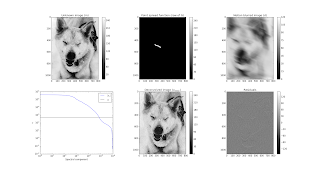### Deconvolution in frequency domain with a few lines of Python codeOriginal image, point spread function that simulates motion blur, convolved image (blurred image), spectral components of the image, deconvolved image, and residuals.
Convolution appears in nearly every measurement problem. A well know example is the Hubble space telescope. In the early days, deconvolution techniques had to be used to correct for a flaw in the telescope's mirror.

Here's a demonstration of deconvolution I wrote.. The point is to demonstrate that a discrete Fourier transform (FFT) can be used to diagonalize a Toeplitz-matrix (convolution operator). This allows us to perform linear least-squares on million by million sized full theory matrices in less than a second. This wouldn't be possible otherwise. It is also possible to regularize the problem by applying regularization to each spectral component of the spectrum of the point-spread function, as the all of the matrices (theory matrix, error covariance matrix, and prior covariance matrix) in the full maximum a posteriori estimator can typically be diagonalized as they are also typically Toeplitz matrices.

I've attached the deconvolution code below. This is essentially what I use to deconvolve range-Doppler ambiguity functions from incoherent scatter radar measurements at Arecibo. Only a few lines of code are used to do most of the work.

 
#!/usr/bin/env python
#
# Image deconvolution using FFT
# (c) 2017 Juha Vierinen
#
import scipy.misc as s
import numpy as n
import matplotlib.pyplot as p

# original image

# FFT true image (m)
H=n.fft.fft2(husky)

# FFT point spread function (first column of theory matrix G)
P=n.fft.fft2(psf)

# simulate measurement (d=Gm + \eta)
# also normalize 2d FFT
# fftshit resolves wrapping of spectral components (0..2pi to -pi..pi)
d=(1.0/(H.shape*H.shape))*n.fft.fftshift(n.fft.ifft2(H*P).real)
# add noise with standard deviation of 0.1
d=d+n.random.randn(d.shape,d.shape)*0.1

# FFT2 measurement
# Use image in husky_conv.png
# U^H d
D=n.fft.fft2(d)

# regularization parameter
# (should be one to two orders of magnitude below the largest spectral component of point-spread function)
alpha = 500.0

# -dampped spectral components,
# -also known as Wiener filtering
# (conj(S)/(|S|^2 + alpha^2)) U^H d
M = (n.conj(P)/(n.abs(P)**2.0 + alpha**2.0))*D

# maximum a posteriori estimate of deconvolved image
# m_map = U (conj(S)/(|S|^2 + alpha^2)) U^H d
m_map=(H.shape*H.shape)*n.fft.fftshift(n.fft.ifft2(M).real)

p.subplot(231)
p.imshow(husky,cmap="gray")
p.colorbar()
p.title("Unknown image (m)")

p.subplot(232)
p.imshow(psf,cmap="gray")
p.title("Point spread function (row of G)")
p.colorbar()

p.subplot(233)
p.imshow(d,cmap="gray",vmin=0,vmax=120)
p.title("Motion blurred image (d)")
p.colorbar()

# sort 2d fourier components by magnitude and convert into 1d vector for plotting.
P2=n.abs(n.copy(P).flatten())
P2=n.sort(P2)[::-1]

p.subplot(234)
p.loglog(P2,label="$|s_i|$")
p.axhline(alpha,label="$\\alpha$",color="black")
p.xlabel("Spectral component")
p.ylabel("$s_i$")
p.legend()

p.subplot(235)
p.imshow(m_map,cmap="gray",vmin=0,vmax=255)
p.title("Deconvolved image ($m_{\mathrm{MAP}}$)")
p.colorbar()

p.subplot(236)
p.imshow(husky-m_map,cmap="gray")
p.title("Residuals")
p.colorbar()

p.show()